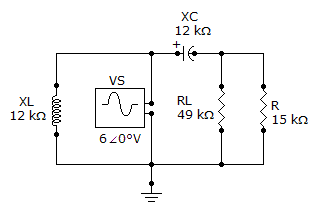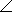# Electrical Engineering - Circuit Theorems in AC Analysis - Discussion

### Discussion :: Circuit Theorems in AC Analysis - General Questions (Q.No.2)

2.

For the given circuit, find VTH for the circuit external to RL.[A]. 4.6951.3° V [B]. 4.6938.7° V [C]. 46938.7° mV [D]. 60° V

Explanation:

No answer description available for this question.

 Biswajit Patra said: (Feb 11, 2014) I want the process to solve this?

 Nikhil Jain said: (Jun 29, 2014) The value of C is j12 kohm and l is j12 kohm. First remove RL and consider vth at the place of Rl then apply nodal analysis. You'll get the answer

 Chinna said: (Aug 25, 2014) Can you please elaborate the explanation by substituting values @Nikhil Jain.

 Sky_Net said: (Dec 5, 2014) Can anybody tell true procedure?

 Annnonymus said: (Sep 24, 2015) Use kcl to find current in 15 kohm resistance. Then find voltage and that will answer.

 Nhuss said: (Jan 30, 2016) XL = 12 K OHMS. XC = 12 K OHMS. RL = 49 K OHMS. R = 15 K OHMS. VS = 6 < 0 V. Z = SQRT ((R+RL)^2 + (XL-XC)^2). Z = R + RL = 49 + 15 = 64 K OHMS. I = V/Z = 6/64K = 0.09375 mA. VTH = 0.09375 mA x 49 k OHMS = 4.59 V.

 Abdi said: (Apr 21, 2016) If you write equation for loop 1(branch with the inductor) and loop 2(the branch with the capacitor and R after removing RL): Loop 1: I1Xl = 6V Loop 2: I2(15000-j12000) = 6 (since we are interested Vth across R, we can find I2, then find the voltage across R. Because Rl is in parallel with R, the voltage across R is the same as Vth) I2 = 6V/(15000-j120000)= 3.1235*10^-4 Vr=Vth = 15000 * 3.1235*10^-4 = 4.685 Hope this helps!

 Hasan said: (May 12, 2016) Eth is the voltage across R resistance. So, Eth = 6*15/(15 - 12j) = 4.68 < 38.7.

 Siddhant Mukherjee said: (Dec 12, 2016) The Vth across RL is equal to the voltage across the resistor R. So for determining the voltage across R we need to find out the current flowing through R. The Current across R would be equal to I (across R) = VS/(R+XC) So the Voltage across R would be equal to IR. Vth = I (across R) * R. = (VS*R)/(R + XC). Put the values of the respective parameters and the answers would be option b = 4.69<38.7° V.

 Kishorvarma said: (Dec 12, 2016) It's very good explanation @Nhuss.

 Azeem said: (Jul 24, 2020) It is very simple. 1) As we know voltage across parallel is same so XL is useless to calculate; 2) To find vth RL is open circuit so remove RL. 3) Now we have VS= 6 V and Xc= 12 K is in series with R = 15 K. 4) Z = (R^2+Xc^2)^1/2 = 19.2 K. 5) Vth = R/Z * Vs = .78*6 = 4.69. 6) θ = tan-1 ( Xc/R) = .6747 rad * 57 = 38.5°.